Product of increasing functions need not be increasing

Statement

It is possible to have two real-valued functions$f,g$, both defined on all real numbers, such that both$f$ and$g$ are increasing functions but the product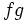$fg$ (defined as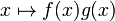$x \mapsto f(x)g(x)$) is not an increasing function on all of$\R$.

We can also adapt this to functions defined on any open interval or closed interval instead of all real numbers.

Proof

Counterexample

Since we need to disprove a general statement, it is enough to exhibit one counterexample.

The example is with$f,g$ both the identity function: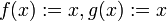$\! f(x) := x, g(x) := x$

These are both increasing on all real numbers.

The product is: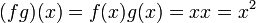$\! (fg)(x) = f(x)g(x) = xx = x^2$

This is the square function which is decreasing on$(-\infty,0)$ and increasing on$(0,\infty)$.

Generic idea behind counterexample

A product of negative increasing functions is decreasing.

Compatibility with product rule for differentiation

The product rule for differentiation states that: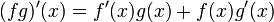$\! (fg)'(x) = f'(x)g(x) + f(x)g'(x)$

In particular, the sign of$(fg)'$ depends not merely on the signs of$f'$ and$g'$ but also on the signs of$f$ and$g$. In particular, if$g$ is negative, then multiplying by a positive$f'$ gives a negative product. This is why$(fg)'$ can be negative even though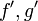$f',g'$ are both positive.